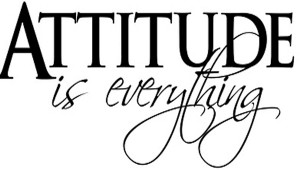reliable source of wise counsel

# For my kids.Thanks to cuz Ahmad Yani for providing me with this. Best status ive read.

If A B C D E F G H I J K L M N O P Q R S T U V W X Y Z were denoted as 1 2 3 4 5 6 7 8 9 10 11 12 13 14 15 16 17 18 19 20 21 22 23 24 25 26

then;

H+A+R+D+W+O+R+K = 8+1+18+4+23+15+18+11 will get you 98% success rate.

K+N+O+W+L+E+D+G+E = 11+14+15+23+12+5+4+7+5 will provide you with 96% success rate.

L+U+C+K = 12+21+3+11 will get you 47% of the time.

However ultimately your;

A+T+T+I+T+U+D+E = 1+20+20+9+20+21+4+5 will ensure 100%.